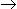# Chemical Engineering - Chemical Reaction Engineering

### Exercise :: Chemical Reaction Engineering - Section 3

41.

Given,
3H2 + CO = CH4 + H2O, KP = 101.84 and,
4H2 + CO2 = CH4 + 2H2O, KP = 101.17
the KP for the reaction CO + H2O = CO2 + H2 is

 A. 103.01 B. 10-0.67 C. 10-3.01 D. l00.67

Explanation:

No answer description available for this question. Let us discuss.

42.

Differential method for analysing the kinetic data is used

 A. for testing complicated mechanisms. B. when the data are scattered. C. when rate expressions are very simple. D. none of these.

Explanation:

No answer description available for this question. Let us discuss.

43.

In case of the irreversible unimolecular type, first order reaction, the fractional conversion at any time for constant volume system as compared to variable volume system is

 A. more B. less C. same D. either (a) or (b), depends on other factors

Explanation:

No answer description available for this question. Let us discuss.

44.

The reaction in which the rate equation corresponds to a stoichiometric equation, is called a/an __________ reaction.

 A. elementary B. non-elementary C. parallel D. autokinetic

Explanation:

No answer description available for this question. Let us discuss.

45.

The reaction AB is conducted in an isothermal batch reactor. If the conversion of A increases linearly with holding time, then the order of the reaction is

 A. 0 B. 1 C. 1.5 D. 2